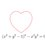# Balance it (if you possibly can)This link came up in a forum. They give you a few weights and a beam balance. The challenge is to put the weights on the balance so as to balance it. (No Pun Intended)

Go and play the game, really.

Now that you've played the game, think of a way to program your way to a solution.

More specifically, the programming problem is this:

You will be given a set of integer such that there is a subset whose sum is exactly half of the sum of the set.

Identify that subset.6 years, 7 months ago

This discussion board is a place to discuss our Daily Challenges and the math and science related to those challenges. Explanations are more than just a solution — they should explain the steps and thinking strategies that you used to obtain the solution. Comments should further the discussion of math and science.

When posting on Brilliant:

• Use the emojis to react to an explanation, whether you're congratulating a job well done , or just really confused .
• Ask specific questions about the challenge or the steps in somebody's explanation. Well-posed questions can add a lot to the discussion, but posting "I don't understand!" doesn't help anyone.
• Try to contribute something new to the discussion, whether it is an extension, generalization or other idea related to the challenge.

MarkdownAppears as
*italics* or _italics_ italics
**bold** or __bold__ bold
- bulleted- list
• bulleted
• list
1. numbered2. list
1. numbered
2. list
Note: you must add a full line of space before and after lists for them to show up correctly
paragraph 1paragraph 2

paragraph 1

paragraph 2

[example link](https://brilliant.org)example link
> This is a quote
This is a quote
    # I indented these lines
# 4 spaces, and now they show
# up as a code block.

print "hello world"
# I indented these lines
# 4 spaces, and now they show
# up as a code block.

print "hello world"
MathAppears as
Remember to wrap math in $$ ... $$ or $ ... $ to ensure proper formatting.
2 \times 3 $2 \times 3$
2^{34} $2^{34}$
a_{i-1} $a_{i-1}$
\frac{2}{3} $\frac{2}{3}$
\sqrt{2} $\sqrt{2}$
\sum_{i=1}^3 $\sum_{i=1}^3$
\sin \theta $\sin \theta$
\boxed{123} $\boxed{123}$

Sort by:

Interesting problem. Here's one solution I can come up with. I would love to see more efficient algorithms in terms of time complexity for this. The style of code is Depth First Search.

Solution in Python

  1 2 3 4 5 6 7 8 9 10 11 12 13 14 15 16 17 18 19 20 21 22 23 def balanceIt(subset1, subset2 = []): ''' Initially all weights are in subset 1 Subsequently weights will be added in subset 2 removing them from subset 1 so that sum of weights in both subsets can be made equal. ''' if sum(subset2) == sum(subset1): return subset1, subset2 if sum(subset2) > sum(subset1): return None for i in subset1: subset1_new = subset1[:] subset1_new.remove(i) result = balanceIt(subset1_new, subset2+[i]) if result != None: return result # Usage: balanceIt(weights) # For instance balanceIt([1,3,2,2]) would return # ([2, 2], [1, 3]) 

- 6 years, 7 months ago

Nice. I'll run this tommorrow and see if I can win the game.

- 6 years, 7 months ago